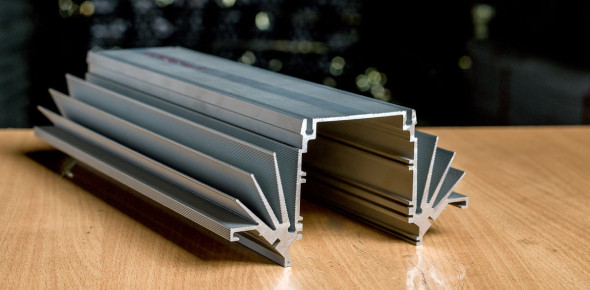Approved & Edited by ProProfs Editorial Team
At ProProfs Quizzes, our dedicated in-house team of experts takes pride in their work. With a sharp eye for detail, they meticulously review each quiz. This ensures that every quiz, taken by over 100 million users, meets our standards of accuracy, clarity, and engagement.
A
Community Contributor
Quizzes Created: 951 | Total Attempts: 894,632
Questions: 10 | Attempts: 203SettingsAluminum is a chemical element which is a silvery-white, nonmagnetic, and ductile metal in the boron group. It is behind oxygen in abundance even though it is less common in the mantle below. Aluminum metal is chemically reactive that native specimens are rare and limited to extreme reducing environments. This short quiz is to test your knowledge as to this chemical element.

• 1.

### Which of the following is the atomic number of Aluminium?

• A.

13

• B.

15

• C.

17

• D.

11

A. 13
Explanation
The atomic number of an element represents the number of protons in the nucleus of an atom of that element. In the case of Aluminium, the atomic number is 13, which means that an atom of Aluminium contains 13 protons.

Rate this question:

• 2.

### By mass, aluminium makes up to how much of the earth's crust?

• A.

8%

• B.

15%

• C.

5%

• D.

10%

A. 8%
Explanation
Aluminium makes up approximately 8% of the earth's crust by mass. This means that out of all the elements present in the earth's crust, aluminium constitutes around 8% of the total mass.

Rate this question:

• 3.

### Amongst the abundant elements, Aluminium is of which position?

• A.

Second

• B.

Fifth

• C.

Third

• D.

Fourth

C. Third
Explanation
Aluminium is the third most abundant element on Earth. It makes up about 8% of the Earth's crust, following oxygen and silicon. This high abundance is due to its strong affinity for oxygen, forming stable compounds like aluminum oxide. Aluminium is widely used in various industries due to its lightweight, corrosion resistance, and excellent conductivity properties.

Rate this question:

• 4.

### Which of the following is the chief ore of aluminium?

• A.

Manganese

• B.

• C.

Anglesite

• D.

Bauxite

D. Bauxite
Explanation
Bauxite is the chief ore of aluminium. It is a sedimentary rock that is rich in aluminium minerals, such as gibbsite, boehmite, and diaspore. Bauxite is formed through the weathering of aluminium-rich rocks over millions of years. It is the primary source of aluminium production worldwide, accounting for over 90% of the global supply. Bauxite is typically found in tropical and subtropical regions, where the weathering process is more prevalent.

Rate this question:

• 5.

### It is found combined in how many different minerals?

• A.

Less than 250

• B.

Over 270

• C.

290

• D.

Over 280

B. Over 270
Explanation
The correct answer is "Over 270". This means that the element in question is found combined in more than 270 different minerals.

Rate this question:

• 6.

### Which of the following is the electrons per shell of Aluminium?

• A.

2, 8, 3

• B.

3,9,4

• C.

2, 9, 5

• D.

1, 8, 3

A. 2, 8, 3
Explanation
The correct answer is 2, 8, 3. This represents the electron configuration of Aluminium. The first shell can hold a maximum of 2 electrons, the second shell can hold a maximum of 8 electrons, and the third shell can hold a maximum of 3 electrons.

Rate this question:

• 7.

### What is the heat of vaporization?

• A.

284 kJ/mol

• B.

10.71 kJ/mol

• C.

198 kJ/mol

• D.

239 kJ/mol

A. 284 kJ/mol
Explanation
The heat of vaporization refers to the amount of heat energy required to convert a substance from a liquid to a gas at a constant temperature and pressure. In this case, the correct answer is 284 kJ/mol, which means that it takes 284 kilojoules of energy to vaporize one mole of the substance.

Rate this question:

• 8.

### Which of the following is the molar heat capacity?

• A.

127 kJ/mol

• B.

933 J/(mol.K)

• C.

24.20 J/(mol.K)

• D.

10.71 kJ/mol

C. 24.20 J/(mol.K)
Explanation
The molar heat capacity is a measure of the amount of heat energy required to raise the temperature of one mole of a substance by one degree Kelvin (or Celsius). The correct answer, 24.20 J/(mol.K), is in the appropriate unit for molar heat capacity. The unit J/(mol.K) indicates that it is the amount of heat energy required to raise the temperature of one mole of the substance by one degree Kelvin. The other options, 127 kJ/mol, 933 J/(mol.K), and 10.71 kJ/mol, are not in the correct unit for molar heat capacity.

Rate this question:

• 9.

### Which of the following is the Pauling scale of aluminium?

• A.

2.56

• B.

1.61

• C.

1.90

• D.

1.85

B. 1.61
Explanation
The Pauling scale is a measure of electronegativity, which indicates the ability of an atom to attract electrons towards itself in a chemical bond. The Pauling scale ranges from 0.7 (for cesium) to 4.0 (for fluorine). Aluminium has an electronegativity value of 1.61 on the Pauling scale, which suggests that it has a moderate ability to attract electrons.

Rate this question:

• 10.

### What is the Van der Waals radius of Aluminium?

• A.

189 pm

• B.

256 pm

• C.

157 pm

• D.

184 pmBack to top